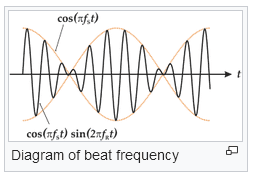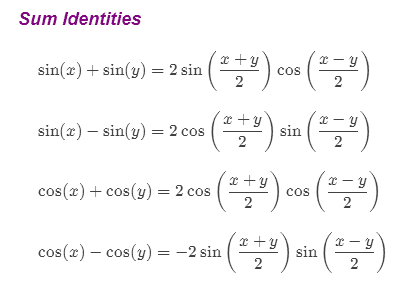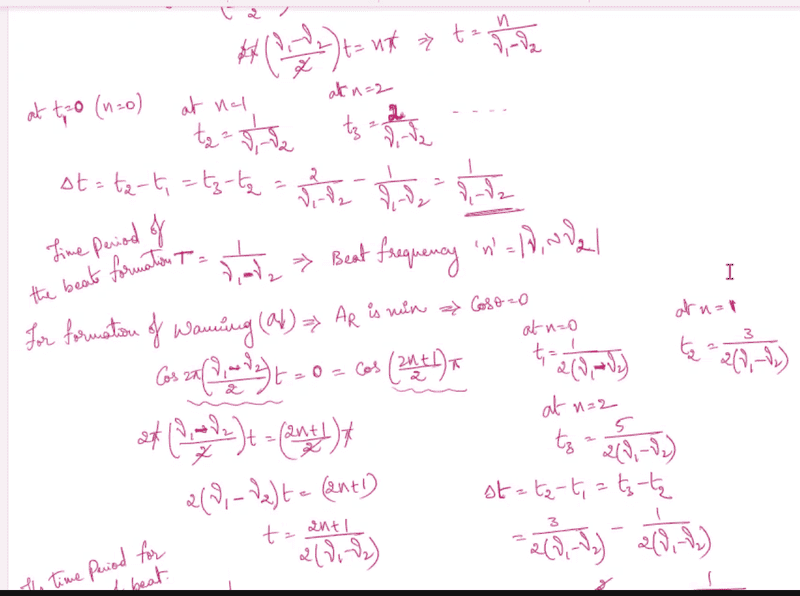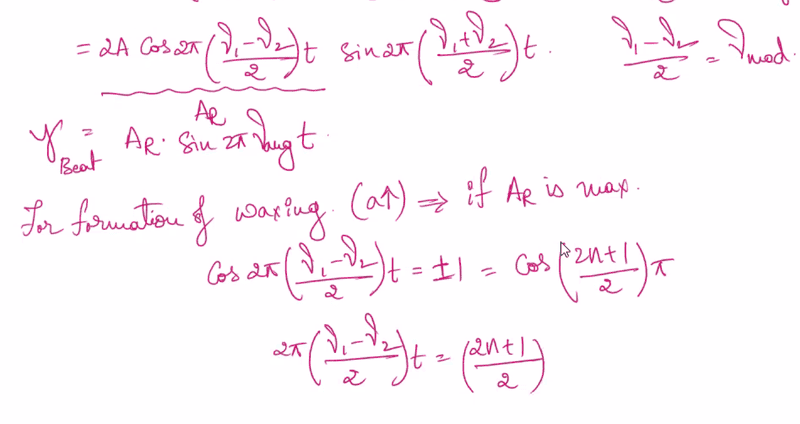# Time period of Beat (acoustics)

• I
• Huzaifa

#### Huzaifa

$$y=2 A \cos 2 \pi\left(\frac{\nu_{1}-\nu_{2}}{2}\right) t \sin 2 \pi\left(\frac{\nu_{1}+\nu_{2}}{2}\right) t$$

Can you explain me the significance of the above equation in the context of waves and oscillations? It's something to do with 'beats,'.

Where did you get it from? They probably derive it from the combination of the two sinusoids at different frequencies, no?

•vanhees71
https://en.wikipedia.org/wiki/Beat_(acoustics)•vanhees71
Where did you get it from? They probably derive it from the combination of the two sinusoids at different frequencies, no?
Yes sir, they derived it from $$y_{1}=A \sin \omega_{1} t ; y_{2}=A \sin \omega_{2} t$$

$$y=y_{1}+y_{2}=A\left[\sin \omega_{1} t+\sin \omega_{2} t\right]=A\left[2 \sin \left(\frac{\omega_{1}+\omega_{2}}{2}\right) t \cos \left(\frac{\omega_{1}-\omega_{2}}{2}\right) t\right]$$

How to proceed ahead from here?

$$y=y_{1}+y_{2}=A\left[\sin \omega_{1} t+\sin \omega_{2} t\right]=A\left[2 \sin \left(\frac{\omega_{1}+\omega_{2}}{2}\right) t \cos \left(\frac{\omega_{1}-\omega_{2}}{2}\right) t\right]$$

How to proceed ahead from here?
Sorry, what do you mean? Are you familiar with common trig identities like the sum of two sine waves?

https://www.purplemath.com/modules/idents.htmConsider two two sinusoids at different frequencies $$y_{1}=A \sin \omega_{1} t ; y_{2}=A \sin \omega_{2} t$$
$$y=y_{1}+y_{2}=A\left[\sin \omega_{1} t+\sin \omega_{2} t\right]=A\left[2 \sin \left(\frac{\omega_{1}+\omega_{2}}{2}\right) t \cos \left(\frac{\omega_{1}-\omega_{2}}{2}\right) t\right]$$

For the formation of waxing, A is maximum, what will be the time period? And for the formation of wanning, A is minimum, what will be the time period?This is from my class notes. Unfortunately, I missed my class and I am not able to understand now. So I have turned to Physics Forums for help.

Please explain me how the time period for the formation of beat T, $$T=\dfrac{1}{\nu_{1}+\nu_{2}}$$
and the beat frequency $$n=\nu_{1} \sim \nu_{2}$$

Sorry, what do you mean? Are you familiar with common trig identities like the sum of two sine waves?

https://www.purplemath.com/modules/idents.htm

View attachment 295097
Yes sir, I know, I solved it, How to solve for time period now? I am not able to understand thisHow to solve for time period now?
Which time period? The sound you hear when listening to audio beats is the sum frequency, modulated at the difference frequency. So the period of the beats (the louder-softer characteristic) is at the difference frequency of the two sine waves.

Which time period? The sound you hear when listening to audio beats is the sum frequency, modulated at the difference frequency. So the period of the beats (the louder-softer characteristic) is at the difference frequency of the two sine waves.
Yes, I think the time period of the beats. Does the time period depend on A? When the A is maximum, $$t=\dfrac{n}{\nu_1-\nu_2}$$, When A is minimum $$t=\dfrac{2n+1}{2 \left( \nu_1-\nu_2 \right)}$$.